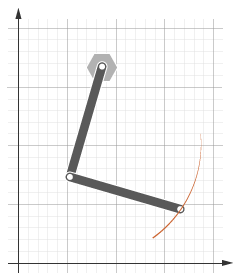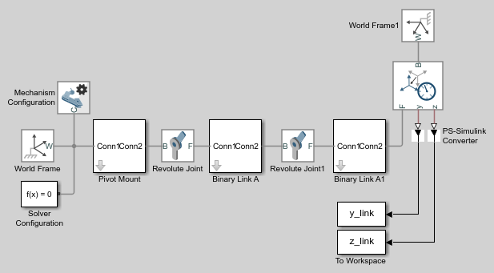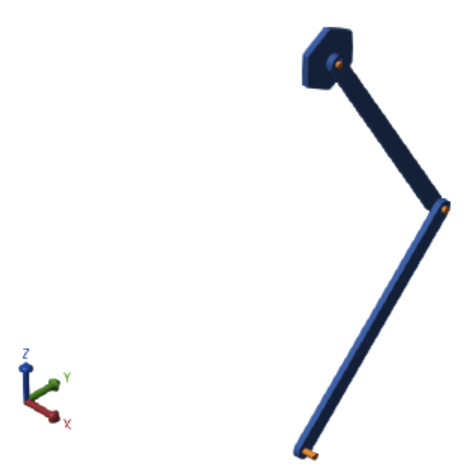## Sense Motion Using a Transform Sensor Block

### Model Overview

The Transform Sensor block provides the broadest motion-sensing capability in Simscape™ Multibody™ models. Using this block, you can sense motion variables between any two frames in a model. These variables can include translational and rotational position, velocity, and acceleration.

In this example, you use a Transform Sensor block to sense the lower link translational position with respect to the World frame. You output the position coordinates directly to the model workspace, and then plot these coordinates using MATLAB® commands. By varying the joint state targets, you can analyze the lower-link motion under quasi-periodic and chaotic conditions.### Modeling Approach

In this example, you rely on gravity to cause the double pendulum to move. You displace the links from equilibrium and then let gravity act on them. To displace the links at time zero, you use the State Targets section of the Revolute Joint block dialog box. You can specify position or velocity. When you are ready, you simulate the model to analyze its motion.

To sense motion, you use the Transform Sensor block. First, you connect the base and follower frame ports to the World Frame and lower link subsystem blocks. By connecting the ports to these blocks, you can sense motion in the lower link with respect to the World frame. Then, you select the translation parameters to sense. By selecting Y and Z, you can sense translation along the Y and Z axes, respectively. You can plot these coordinates with respect to each other and analyze the motion that they reveal.

### Build Model

1. At the MATLAB command prompt, enter `smdoc_double_pendulum`. A double pendulum model opens up. For instructions on how to create this model, see Model an Open-Loop Kinematic Chain.

2. Drag these blocks into the model to sense motion.

LibraryBlockQuantity
Simscape > Multibody > Frames and TransformsTransform Sensor1
Simscape > Multibody > Frames and TransformsWorld Frame1

3. In the Transform Sensor block dialog box, select Translation > Y and Translation > Z. The block exposes two physical signal output ports, labeled y and z.

4. In the PS-Simulink Converter blocks, specify units of `cm`.

5. In the To Workspace blocks, enter the variable names `y_link` and `z_link`.

6. Connect the blocks to the model as shown in the figure. You must connect the base frame port of the Transform Sensor block to the World Frame block. The new blocks are shaded gray.### Guide Model Assembly

Specify the initial state of each joint. Later, you can modify this state to explore different motion types. For the first iteration, rotate only the top link by a small angle.

1. In the Revolute Joint block dialog box, select State Targets > Specify Position Target.

2. Set Value to `10 deg`.

3. In the Revolute Joint1 block dialog box, check that State Targets > Specify Position Target is cleared.

### Simulate Model

Run the simulation. Mechanics Explorer plays a physics-based animation of the double pendulum assembly.You can now plot the position coordinates of the lower link. To do this, at the MATLAB command line, enter:

```figure; plot(y_link.data, z_link.data, 'color', [60 100 175]/255); xlabel('Y Coordinate (cm)'); ylabel('Z Coordinate (cm)'); grid on;```

The figure shows the plot that opens. This plot shows that the lower link path varies only slightly with each oscillation. This behavior is characteristic of quasi-periodic systems.#### Simulate Chaotic Motion

By adjusting the revolute joint state targets, you can simulate the model under chaotic conditions. One way to obtain chaotic motion is to rotate the top revolute joint by a large angle. To do this, in the Revolute Joint dialog box, change State Targets > Position > Value to `90` and click OK.

Simulate the model with the new joint state target. To plot the position coordinates of the lower pendulum link with respect to the world frame, at the MATLAB command prompt, enter this code:

```figure; plot(y_link.data, z_link.data, 'color', [60 100 175]/255); xlabel('Y Coordinate (cm)'); ylabel('Z Coordinate (cm)'); grid on;```

The figure shows the plot that opens.### Save Model

Save the model in a convenient folder under the name `double_pendulum_sensing`. You reuse this model in a subsequent tutorial, Specify Joint Motion in Planar Manipulator Model.